# Integrating factor technique

### Linear equations method of integrating factors

For the past sections we have been studying ways to solve linear first order differential equations with methods such as separable equations, or exact equations, but remember these two methods only work under certain ideal conditions. Therefore, is time for us to study a technique that, although it can be lengthy, is an effective path to solve those problems which do not fit into the first two cases mentioned.

Just as before, we start with a differential equation of the form: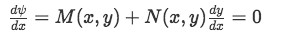Equation 1: General form of first order differential equation

How do we decide which method to use? Well let us talk about the conditions that have to be met for the techniques we have already seen.

For the method of separable equations we can only find the solution to the differential equation if we are able to "separate" all of the x terms from the y terms. In other words, we can only use this technique if we can rearrange all of the terms in the equation in a way that all the x terms end up in one side of the equal sign and all of the y terms are in the other side. If we are able to come up with such "nice" arrangement, the only thing left to do is to directly integrate each side of the equation to easily find the solution. As you already know, this is not always possible, and so we continue to the next best method which is to check for exact differential equations.

In order to use the method of exact equations, we need to assess if the solution is a linear equation that will result in a constant. To do so, we need to identify the terms of M and the terms of Y, and take the partial derivative of M with respect of y, and the partial derivative of N with respect of x and then compare these results. If they are equal, it means this first order differential equation is exact, and we can follow through this technique to find the solution.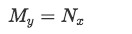Equation 2: Condition for an exact differential equation

But what if $M_{y}$ is not equal to $N_{x}$? What do we do now? Well, this is how we end up with the integrating factor technique. As mentioned before, although effective, the integrating factor method is not the most efficient since it takes longer time and requires a more robust set of calculations and so, we prefer to leave this method as a last resort in solving linear first order differential equations. We recommend that if you have a differential equation to solve, check to see if you can solve it with the two simpler methods first to save time.

The basic principle behind the method of integrating factors is the consistency of proportionality in a mathematical equality. In simpler words, as long as you multiply the whole equation (both sides of the mathematical equality) by a term, the proportionality of the equation remains. This means that if variables are involved in such equation, the values of the variables remain the same since you are applying the changing factor to both the variable and their value by applying it to the whole equation.

For example, if we apply a factor of (x+1) to the original equation of 2x=8 (where is easy to see that x=4), we obtain: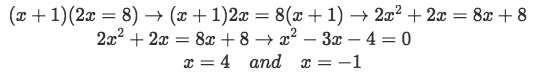Equation 3: Example of proportionality consistency in math equalities

Notice that the value of x=4 remained, even though the inserted factor gave another possible value to the variable, the original equality remained.

And so we use this basis to multiply our given first order differential equation by a function of x we call the integrating factor (or integration factor) so we can convert the differential equation into an exact one and continue the solution of the problem in a manner we already know.

Take note that when multiplying this new unknown function to the whole equation we usually define this new function as a function of x. Truthfully speaking, we use a function of x as our integrating factor standard for practical purposes; you could very well use a function of y or a function of both x and y as the integrating factor, but there are two reasons why using a function of x is more convenient: first of all, using a function of two variables (a function of both x and y) as your integrating factor will highly complicate the problem. Second, although using a function in terms of y sounds as simple as using one for x, do not forget y is a dependent variable defined in terms of x and so, by using an integrating factor without y terms we are following the simplest path to continue towards a solution for the differential equation.

Before we start the math on how to solve integrating factor differential equations it is worth mentioning that this article will explain methodology following the assumption that you will use this technique as a last resort. This means that we will check the differential equation given in each problem to see if it is solvable by either separable or exact equations before we try to use an integrating factor, and so, although a general integrating factor formula exists to solve first order differential equations, we will not use it to focus on such cases in which we apply other conditions to find our factor.

For a step by step derivation of the general formula for integrating factors you can visit these differential equation notes and follow the process yourself. We will leave this as independent study for you and recommend you to check out the examples on solving differential equations in the form y'+Py=Q found on the attached link.

## How to find integrating factor for non exact equations

Let us work through our first example to learn how to find the integrating factor. For this first problem we will solve the differential equation by finding its function solution but we will not be applying an initial condition yet. So this will serve just to show the process of finding an equation solution, we will go through a second and third example later, on which we will use a condition to find the explicit solution.

Example 1

Find the general solution of the differential equation: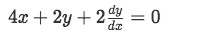Example 1: Solve the given differential equation
• We first check for the possibility of finding the solution through the exact equations method. So we identify M and N for the equation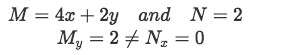Example 1(a): identifying M and N and checking if equation is exact
• As you can see the equation given is not an exact equation, so we apply an integrating factor and identify the new M and N: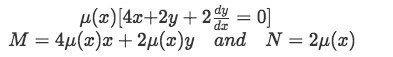Example 1(b): Applying the integrating factor and identifying the new M and N
• Now we set the equation to be exact, by "forcing" the partial derivative of new M with respect to y to be equal to the partial derivative of the new N with respect to x.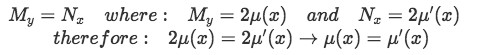Example 1(c): Setting the equation as exact to find the integrating factor
• We use this condition to find the integrating factor: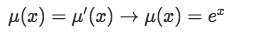Example 1(d): Finding the integrating factor
• So we rewrite the equation given with the integrating factor value found: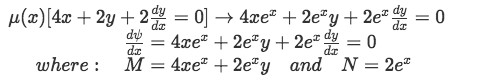Example 1(e): Rewriting the differential equation with the value found for the integrating factor
• Now we solve through the method of exact equations. Remember that this new equation is already known to be exact since we forced this condition in the equations for example 1(c), therefore we skip the "checking if the equation is exact" part and continue on to integrating either M or N to find Psi. For this case, we select to integrate N in order to find Psi: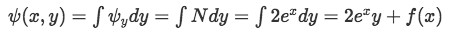Example 1(f): Integrating N to find Psi
• We partially derive this newly found Psi with respect to x, remember this partial derivation is equal to M and so we use this equality to find the value for the function f(x) at the end of the expression.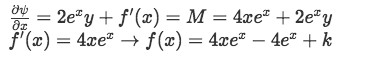Example 1(g): Finding function f(x)
• Notice that we found f(x) by integrating the expression found for f'(x). Having found f(x) we can rewrite the whole expression for Psi: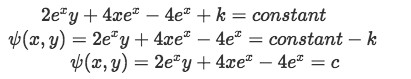Example 1(h): Finding the final general solution Psi

Observe how we played around with the constants at the end. We could easily just dismiss the constant k from the equation in example 1(g) but we decided to keep it so you can observe why we could just dismiss it. Simply explained: the general expression for Psi is equal to an unknown constant (you won't know this constant unless you apply an initial condition) and so, k is also an unknown constant, if you just pass it to the right hand side and group it with the constant already there you find a new value which is just another constant (which we called c) and so we simplify the outcome by just writing this c at the end.

After working on this example, let us list the steps needed to go through the complete method of differential equations integrating factor:

1. First check for the possibility of finding the solution through the exact equations method.
1. Identify M and N.
2. Check if the partial derivative of M with respect to y is equal to the partial derivative of N with respect to x.
3. If $M_{y} = N_{x}$, solve the problem using exact equations method. If they are different, find the integrating factor.
2. Apply an integrating factor and identify the new M and N.
3. Set the new equation to be exact, by setting the partial derivative of new M with respect to y equal to the partial derivative of the new N with respect to x.
4. Find the value of the integrating factor using the equality from step 3. This will usually require an integration.
5. Rewrite the differential equation with the value found for the integrating factor and identify the new M and N. This new equation is exact (since we forced it to be in step 3).
6. Integrate either M or N to find Psi.
1. If you pick M, integrate with respect to x. The result will contain a last term which will be a function in terms of y.
2. If you pick N, integrate with respect to y. The result will contain a last term which will be a function in terms of x.
7. Partially derive the newly found Psi to find the value of the function at the end.
1. If you followed step 6.a then you will have to partially derivative Psi with respect to y. The resulting expression will be equal to N and you will be able to obtain the value of f(y).
2. If you followed step 6.b then you will have to partially derivative Psi with respect to x. The resulting expression will be equal to M and you will be able to obtain the value of f(x).
8. Having found the end function you can rewrite the whole expression for Psi.
9. Apply the initial conditions (if given, if not your are done) to find the explicit solution of Psi.

Now you should be able to work through problems using this technique in a simple way!

## Integrating factor differential equation examples

Having summarized the steps to work through the first order differential equation integrating factor method, we would like to finalize this lesson by solving differential equations in a second and third integrating factor example.

Example 2

Find an integrating factor and solve the given equation having the initial condition $y(1)=\frac{1}{2}$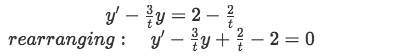Example 2: Solve the given differential equation

Notice that the equation given is in terms of t and y, not in x and y.

• Step 1:

Is the given equation exact? We identify M and N and check for the condition $M_{y} = N_{t}$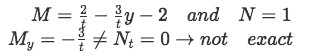Example 2(a): identifying M and N and checking if equation is exact
• Step 2:

Now we apply an integrating factor to the equation and identify the new M and N: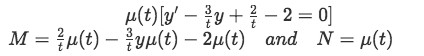Example 2(b): Applying the integrating factor and identifying the new M and N
• Step 3:

Set this new equation to be exact. Thus we set the partial derivative of new M with respect to y equal to the partial derivative of the new N with respect to t.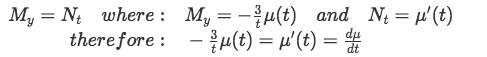Example 2(c): Setting the equation as exact to find the integrating factor
• Step 4:

Find the value of the integrating factor using the equality from the last step and the method of separable equations: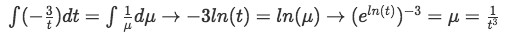Example 2(d): Finding the integrating factor
• Step 5:

Now, let us rewrite the differential equation with the value found for the integrating factor and identify the new M and N. Do not forget this new equation is exact (since we forced it to be in step 3).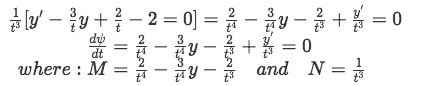Example 2(e): Rewriting the differential equation with the value found for the integrating factor
• Step 6:

Integrate N with respect to y to find Psi.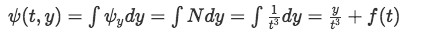Example 2(f): Integrating N to find Psi
• Step 7:

Partially derive the newly found Psi with respect to t in order to find the value of the function at the end.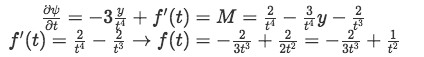Example 2(g): Finding function f(t)
• Step 8:

We can now rewrite the whole expression for Psi.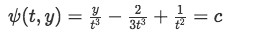Example 2(h): Finding the general solution of Psi
• Step 9:

Apply the initial condition $y(1)=\frac{1}{2}$ to find the explicit solution of Psi.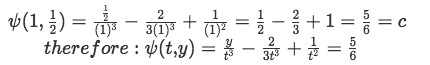Example 2(i): Explicit solution of Psi

Example 3

For our last example we will solve the following equation with the initial condition $y(1)= \frac{1}{2}$.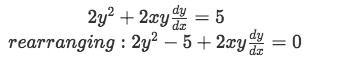Example 3: Solve the given differential equation

We are back to having an equation in terms of x and y. Remember the names of the variables in an equation can be anything, and so, just work with whichever letters the problem requires. If you have a variable named y this will usually be the dependent variable and therefore it doesn't matter the name of the other one, that will be the independent variable (the one you write first). For example, in the problem for example 2 the variables where t,y meaning t was the independent variable (t could be written in terms of y).

• Step 1:

First we identify M and N and check for the condition $M_{y} = N_{t}$ to see if the equation is exact: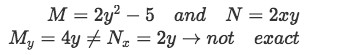Example 3(a): identifying M and N and checking if equation is exact
• Step 2:

Now we apply an integrating factor to the equation and identify the new M and N: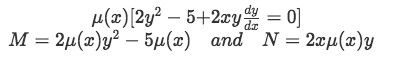Example 3(b): Applying the integrating factor and identifying the new M and N
• Step 3:

Set this new equation to be exact. Thus we set the partial derivative of new M with respect to y equal to the partial derivative of the new N with respect to t.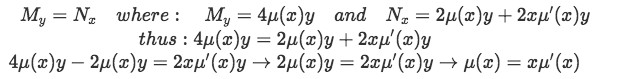Example 3(c): Setting the equation as exact to find the integrating factor
• Step 4:

Through the method of separable equations, find the value of the integrating factor using the last equality from the last step: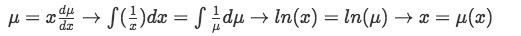Example 3(d): Finding the integrating factor
• Step 5:

We can now rewrite the differential equation (now exact) with the value found for the integrating factor and identify the new M and N.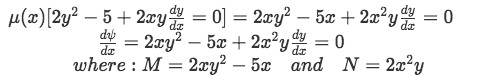Example 3(e): Rewriting the differential equation with the value found for the integrating factor
• Step 6:

Integrate M with respect to x to find Psi.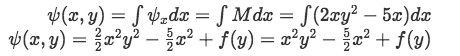Example 3(f): Integrating N to find Psi
• Step 7:

We now partially derive the newly found Psi with respect to y in order to find the value of the function at the end.Example 3(g): Finding function f(t)
• Step 8:

We can now rewrite the whole expression for Psi.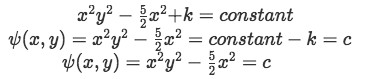Example 3(h): Finding the general solution of Psi
• Step 9:

Apply the initial condition $y(1)=\frac{1}{2}$ to find the explicit solution of Psi.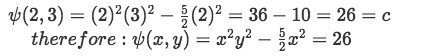Example 3(i): Explicit solution of Psi

For further reading on the integrating factor method we suggest you to take a look at this tutorial module on how to solve first order linear differential equations through this technique.

### Integrating factor technique

#### Lessons

Let's suppose we wish to solve a differential problem of the form:

$M(x,y)+N(x,y) \frac{dy}{dx}=0$

But we cannot do the separable equations, and also $M_y \neq N_x$, so we cannot use exact equations.

But what if we could multiply the whole differential equation by some new equation that would make this problem exact? Let's suppose there exists some sort of function that can do this trick. This function could be a function of $x$ or possibly some function of $y$. Let's suppose that the function that does this trick is $\mu(x)$.

$\mu(x)*[M(x,y)+N(x,y) \frac{dy}{dx}=0]$

$\Longrightarrow \mu(x)M(x,y)+\mu(x)N(x,y) \frac{dy}{dx}=0$

And the whole goal of this is to have $[\mu(x)M(x,y) ]_y=[\mu(x)N(x,y) ]_x$, so we can use our Exact Equations Method.

So if we can choose a $\mu(x)$ such that $[\mu(x)M(x,y) ]_y=[\mu(x)N(x,y) ]_x$ then choose this $\mu(x)$ and multiply the original equation by it:

$\mu(x)M(x,y)+\mu(x)N(x,y) \frac{dy}{dx}=0$

Now just solve this using the Exact Equation Method.

As we multiplied the entire equation by $\mu(x)$ every solution to

Where $\mu(x)\neq 0$ will also be a solution to

$M(x,y)+N(x,y) \frac{dy}{dx}=0$

Which was our original problem.

e.g. If we had an equation $2x=10$ and multiplied the whole equation by $2x+1$ (which could be our $\mu(x)$, then we will have

$2x(2x+1)=10(2x+1)$

$4x^2+2x=20x+10$

Note how $x=5$ is a solution to both $2x=10$ and $4x^2+2x=20x+10$. The only extra solution we picked up was $x=-\frac{1}{2}$, which is the case where $\mu(x)=0$.
• Introduction
What is the Integrating Factor Technique?

• 1.
Solving Differential Equation Using the Integrating Factor Technique
Solve the following differential equation:

$4x+2y+2 \frac{dy}{dx}=0$

• 2.
Find a solution to the following first-order differential equation:

$y'-\frac{3}{t} y=2- \frac{2}{t}$

With initial values $y(1)=\frac{1}{2}$